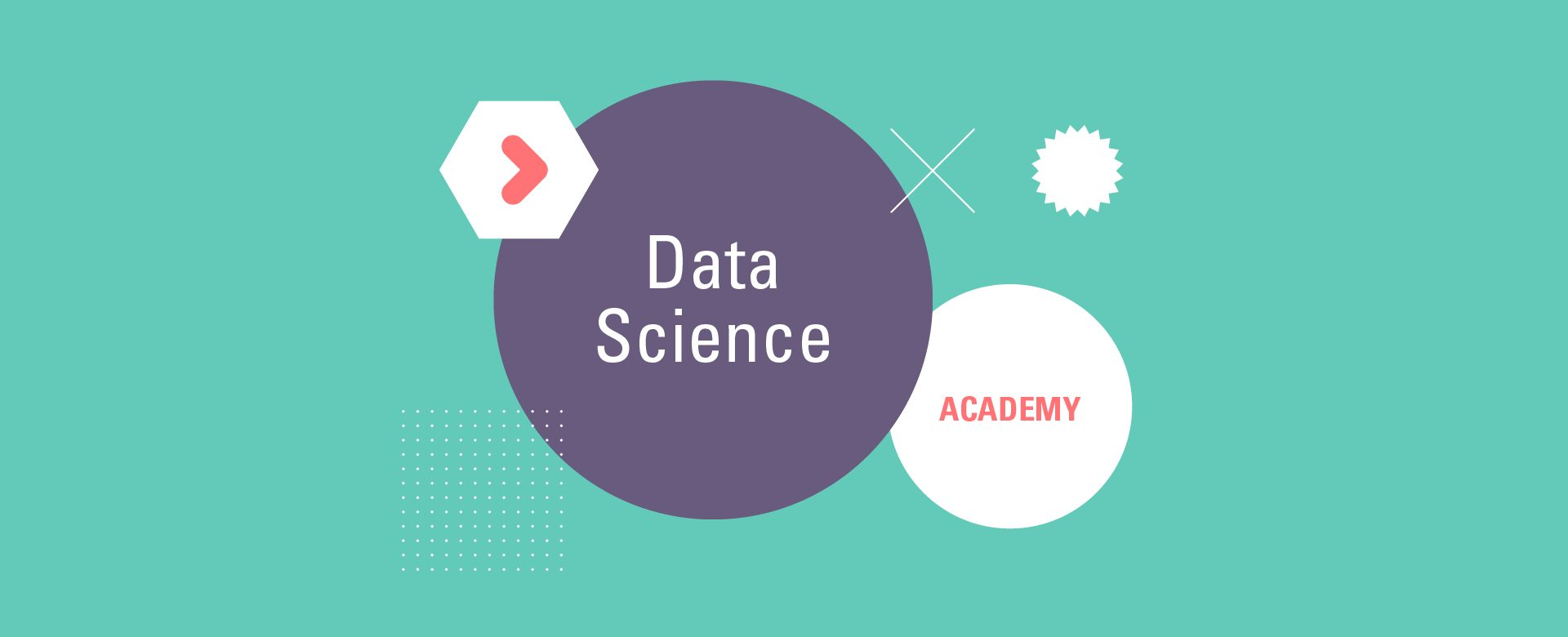# Data Science Fundamentals

€ 1.295,-

The basic concepts of data science and machine learning will be introduced during this training. We start with theory but will quickly put that theory into practice. First, with drag-and-drop no-code tools, and later in the course, we will train machine learning models with the programming language Python.

## Introduction Data Science Fundamentals

The basic concepts of data science and machine learning will be introduced during this training. We start with theory but will quickly put that theory into practice. First, with drag-n-drop no-code tools, and later in the course, we will train machine learning with the programming language Python. At the end of the training, the participants will understand the basic concepts and potential of data science and the way machine learning models are trained and used.

## Main Subjects

The main subjects we will cover in this training are:

• Data Science theory
• Python basics
• Machine learning concepts
• How to get a Data Science project up and running

## Full Program

Day 1

 09.00 – 10.00 – Introduction – Theory 10.00 – 11.30 – Data Science theory – Theory 11.30 – 12.00 – Introduction to KNIME – Theory 12.30 – 14.30 – No-Code Machine learning – Theory 14.30 – 16.30 – Introduction to Python – Practice

Day 2

 09.00 – 09.30 – Recap day 1 – Theory 09.30 – 10.00 – Discuss Python Homework Practice 10.00 – 17.00 – Data Science in Python part 1 Practice

Day 3

 09.00 – 09.30 – Recap day 2 – Theory 09.30 – 10.00 – Discuss Python Homework Practice 10.00 – 12.30 – Data Science in Python part 2 Practice 12.30 – 16.00 – Data Science Hackathon Practice

xxxx